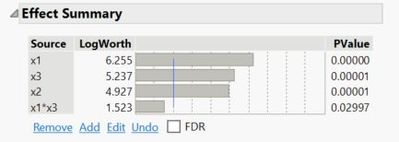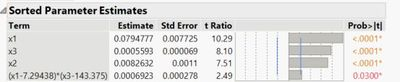Our World Statistics Day conversations have been a great reminder of how much statistics can inform our lives. Do you have an example of how statistics has made a difference in your life? Share your story with the Community!
Choose Language Hide Translation Bar
Highlighted

## LogWorth value in the "Sorted Parameter Estimates" Table

Dear all,

I wanted to ask about the PValue of the LogWorth in the "Sorted Parameter Estimates" report.

The LogWorth in the "Effect Summary" report is defined at the .01 significance level (Pvalue = .01 -> LogWorth = 2).

I've added an example bellow. In the "Sorted Parameter Estimates", you can clearly see that the blue line does not cross the bars at the same level as in the "Effect Summary". I am wondering if it is set at .05 as LogWorth (PValue .05) is 1.3.

Other questions :

- Is there anyway to set a LogWorth at a PValue of .05 ?

- Is there anyway to hide the mean value for factor given in the table "Sorted Parameter Estimates"  ?

Thanks a lot!

Islem2 ACCEPTED SOLUTIONS

Accepted Solutions
Highlighted

## Re: LogWorth value in the "Sorted Parameter Estimates" Table

The Effect Summary table does indeed draw the line at a LogWorth of 2, which corresponds to a alpha value of 0.01.

The Sorted Parameter Estimates table draws that blue line at alpha= 0.05, which is a LogWorth of approximately 1.3.

I do not know of any way to change where the blue indicator lines are drawn.

When you ask if you can hide the "mean value" in the Sorted Parameter Estimates, are you referring to the blue line at 0 in the small plot? If so, the answer is no, I don't think that can be removed. Remember that parameter estimates can be positive and/or negative. Since this graph would show negative bars off to the left, you need that reference of 0. You can hide that graph, but I don't see any easy way to manipulate the elements in that plot.

Dan Obermiller
Highlighted

## Re: LogWorth value in the "Sorted Parameter Estimates" Table

Ah, yes. I believe you also asked this question in another thread. The centering is done to reduce the collinearity that is caused by scale differences. It is a way of JMP helping you with your analysis.

For example, suppose factor X1 has the range 100 to 200 and factor X2 has the range 1 to 2 with this design:

X1    X2     X1*X2

100   1         100

100   2         200

200   1         200

200   2         400

The interaction would have the range 100 to 400. The correlation between X1 and the X1*X2 interaction is 0.688 which is quite high. If I center X1 and X2 before multiplying, the correlation between X1 and the centered X1*X2 interaction is 0. This is a good thing which is why JMP does it. Further, JMP provides the tools needed to understand the model and evaluate it with the centering in place.

Can you turn this off? Yes. In the Fit Model dialog box, click the red triangle in the upper left and "uncheck" the Center Polynomials choice before clicking Run. However, keep in mind that by doing this you will be increasing the multicollinearity in the model which, in turn, increases the variance of parameter estimates and affects the statistical testing and significance for those parameter estimates.

Dan Obermiller
4 REPLIES 4
Highlighted

## Re: LogWorth value in the "Sorted Parameter Estimates" Table

The Effect Summary table does indeed draw the line at a LogWorth of 2, which corresponds to a alpha value of 0.01.

The Sorted Parameter Estimates table draws that blue line at alpha= 0.05, which is a LogWorth of approximately 1.3.

I do not know of any way to change where the blue indicator lines are drawn.

When you ask if you can hide the "mean value" in the Sorted Parameter Estimates, are you referring to the blue line at 0 in the small plot? If so, the answer is no, I don't think that can be removed. Remember that parameter estimates can be positive and/or negative. Since this graph would show negative bars off to the left, you need that reference of 0. You can hide that graph, but I don't see any easy way to manipulate the elements in that plot.

Dan Obermiller
Highlighted

## Re: LogWorth value in the "Sorted Parameter Estimates" Table

Thanks a lot for your answer regarding LogWorth and alpha values, it is more clear for me now.

For the 3rd question, what I wanted to say is that for the interaction between factors we have :

- In the "Effect Summary" : x1*x3

- In the "Sorted Parameter Estimates" : (x1 -7.3) * (x3 -143.4). For your information, 7.3 and 143.3 are the mean values for x1 and x3, respectively.

I didn't understand what (x1 -7.3) * (x3 -143.4) refers to. Why it is not x1*x3 as in the "Effect Summary" ?

I hope it is clearer now.

Highlighted

## Re: LogWorth value in the "Sorted Parameter Estimates" Table

Ah, yes. I believe you also asked this question in another thread. The centering is done to reduce the collinearity that is caused by scale differences. It is a way of JMP helping you with your analysis.

For example, suppose factor X1 has the range 100 to 200 and factor X2 has the range 1 to 2 with this design:

X1    X2     X1*X2

100   1         100

100   2         200

200   1         200

200   2         400

The interaction would have the range 100 to 400. The correlation between X1 and the X1*X2 interaction is 0.688 which is quite high. If I center X1 and X2 before multiplying, the correlation between X1 and the centered X1*X2 interaction is 0. This is a good thing which is why JMP does it. Further, JMP provides the tools needed to understand the model and evaluate it with the centering in place.

Can you turn this off? Yes. In the Fit Model dialog box, click the red triangle in the upper left and "uncheck" the Center Polynomials choice before clicking Run. However, keep in mind that by doing this you will be increasing the multicollinearity in the model which, in turn, increases the variance of parameter estimates and affects the statistical testing and significance for those parameter estimates.

Dan Obermiller
Highlighted

## Re: LogWorth value in the "Sorted Parameter Estimates" Table

Very well explained ! Thanks a lot.
Article Labels